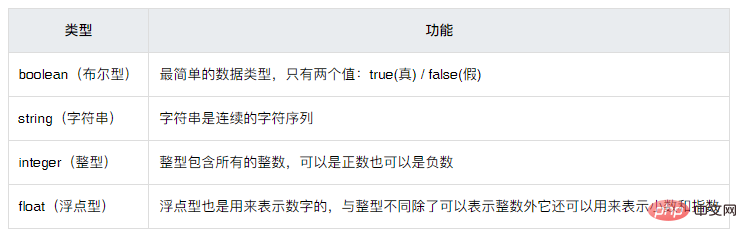# 数组是php中的标量类型吗## 数组是php中的标量类型吗

PHP 的数据类型可以分为三大类，分别是基本数据类型（标量数据类型）、复合数据类型和特殊数据类型。1、布尔型（boolean）

2、字符串型（string）

```<?php
//双引号方式声明字符串
\$str1 = "PHP中文网";
//单引号方式声明字符串
\$str2 = 'PHP 教程';
//Heredoc 方式声明字符串
\$str3 = <<<EOF
url：
https://www.php.cn/
EOF;
echo \$str1."<br>".\$str2."<br>".\$str3;
?>```

```PHP中文网
PHP 教程
url： https://www.php.cn/```

3、整型（integer）

```<?php
\$x = 5985;      // 定义一个整型数据类型的变量
var_dump(\$x);   // 输出此变量
echo "<br>";
\$x = -345;
var_dump(\$x);
echo "<br>";
\$x = 0x8C;      //十六进制数字
var_dump(\$x);
echo "<br>";
\$x = 047;       //八进制数字
var_dump(\$x);
?>```

```int(5985)
int(-345)
int(140)
int(39)```

4、浮点型（float）

```<?php
\$num1 = 10.365;
\$num2 = 2.4e3;
\$num3 = 8E-5;
var_dump(\$num1, \$num2, \$num3);
?>```

`float(10.365) float(2400) float(8.0E-5)`• 相关标签：php
• 相关文章

相关视频